How is the calculator picking the "best" regression line? MathBitsNotebook.com Terms of Use   Contact Person: Donna Roberts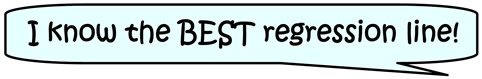Is the calculator checking residuals on EVERY possible regression line to find the least squares line (the best fitting line)? After all, by definition, the least squares line is the line which has the smallest sum of the squares of the residuals.
Well, the answer is "probably not"! How would the calculator know that it had checked a sufficient number of lines and residuals to have arrived at the absolute best choice?

It is more likely that your calculator is using a formula for the best fit regression line, also called the least squares line. Don't get too excited that you have discovered a short cut to finding the best regression lines! Such formulas can be rather messy.

Here is the formula for a linear least squares line, or best fit straight line:
(Remember that the equation of a line is y = mx + b, with m = slope and b = y-intercept.)
 A least squares line associated with a scatter plot of n points: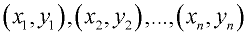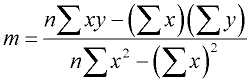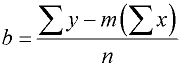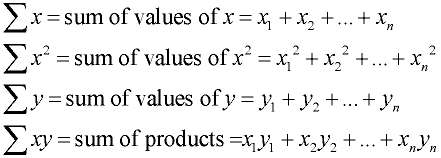Isn't it grand that the calculator does all of this work and gives us, in a manner of seconds, a regression equation that is the best fitting regression equation!